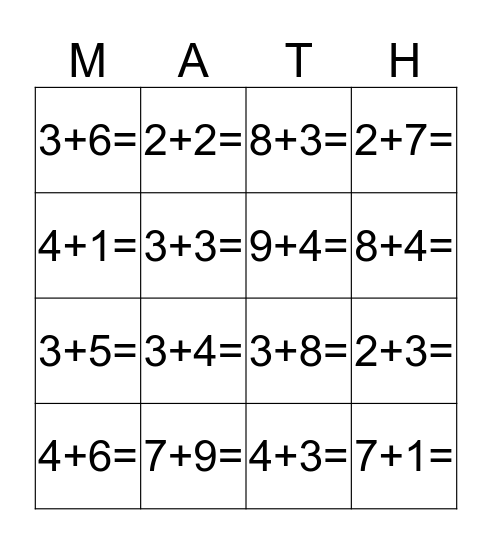This bingo card has 72 words: 2+1=, 2+2=, 2+3=, 2+4=, 2+5=, 2+6=, 2+7=, 2+8=, 3+5=, 2+9=, 3+1=, 3+2=, 3+4=, 3+6=, 3+7=, 3+8=, 3+9=, 4+1=, 4+2=, 4+3=, 4+5=, 4+6=, 4+7=, 4+8=, 4+9=, 5+1=, 5+2=, 5+3=, 5+4=, 5+6=, 5+7=, 5+8=, 5+9=, 6+1=, 6+2=, 6+3=, 6+4=, 6+5=, 3+3=, 4+4=, 5+5=, 6+6=, 6+7=, 6+8=, 6+9=, 7+1=, 7+2=, 7+3=, 7+4=, 7+5=, 7+6=, 7+7=, 7+8=, 7+9=, 8+1=, 8+2=, 8+3=, 8+4=, 8+5=, 8+6=, 8+7=, 8+8=, 8+9=, 9+1=, 9+2=, 9+3=, 9+4=, 9+5=, 9+6=, 9+7=, 9+8= and 9+9=.

## Play Online